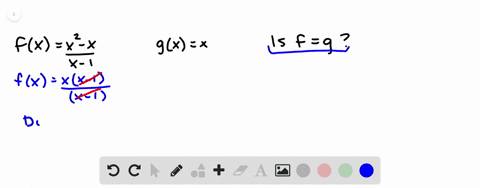Enroll in one of our FREE online STEM summer camps. Space is limited so join now!View Summer Courses### If $f(x) = \frac{x^2-x}{x-1}$ and $g(x) = x$ …

01:38Oregon State University

Need more help? Fill out this quick form to get professional live tutoring.

Get live tutoring
Problem 1

If $f(x) = x + \sqrt{2-x}$ and $g(u) = u + \sqrt{2-u}$, is it true that $f = g$?

True.

## Discussion

You must be signed in to discuss.
Xingtao Y.
December 16, 2017
What if u is equal to one over x?
Sermera D.
June 29, 2020
All of these questions are answered for me... IS there anyway for me to answer myself?

## Video Transcript

when you have functions like F of X and G of axe and H of X, and so on. The letter you choose to use for the input is really arbitrary. There's nothing sacred about using X. There's nothing sacred about using you. So this function here, which is currently called F of X. It's the same function if I call it f of you. And if I replace all the exes with use, it means the exact same thing. And this function g over here, it's the exact same function. If I replace all the use with exes, so are these the same function G of X equals X plus two plus the square to minus U f of X equals X plus square to minus you. Yes, those are identical.